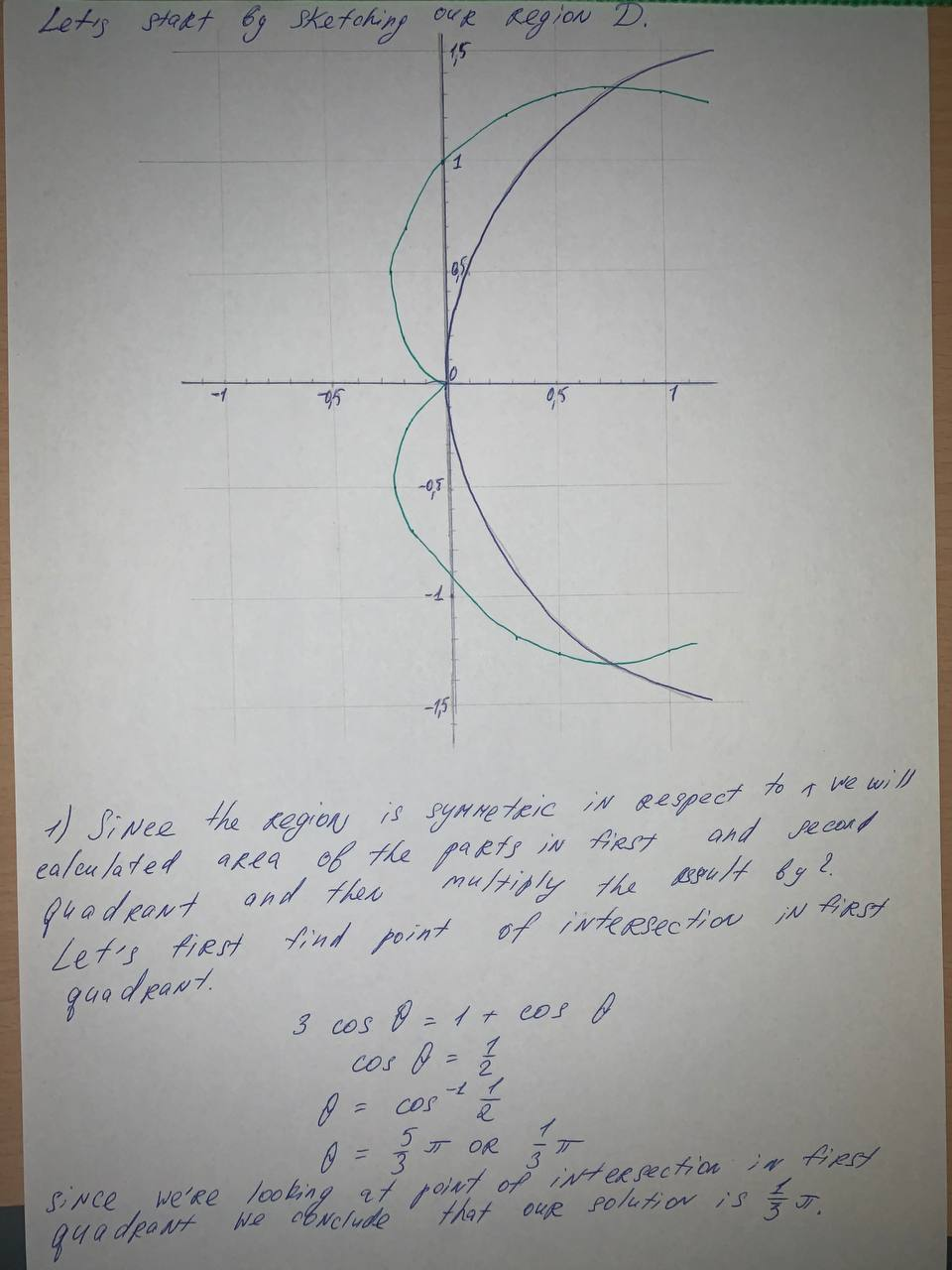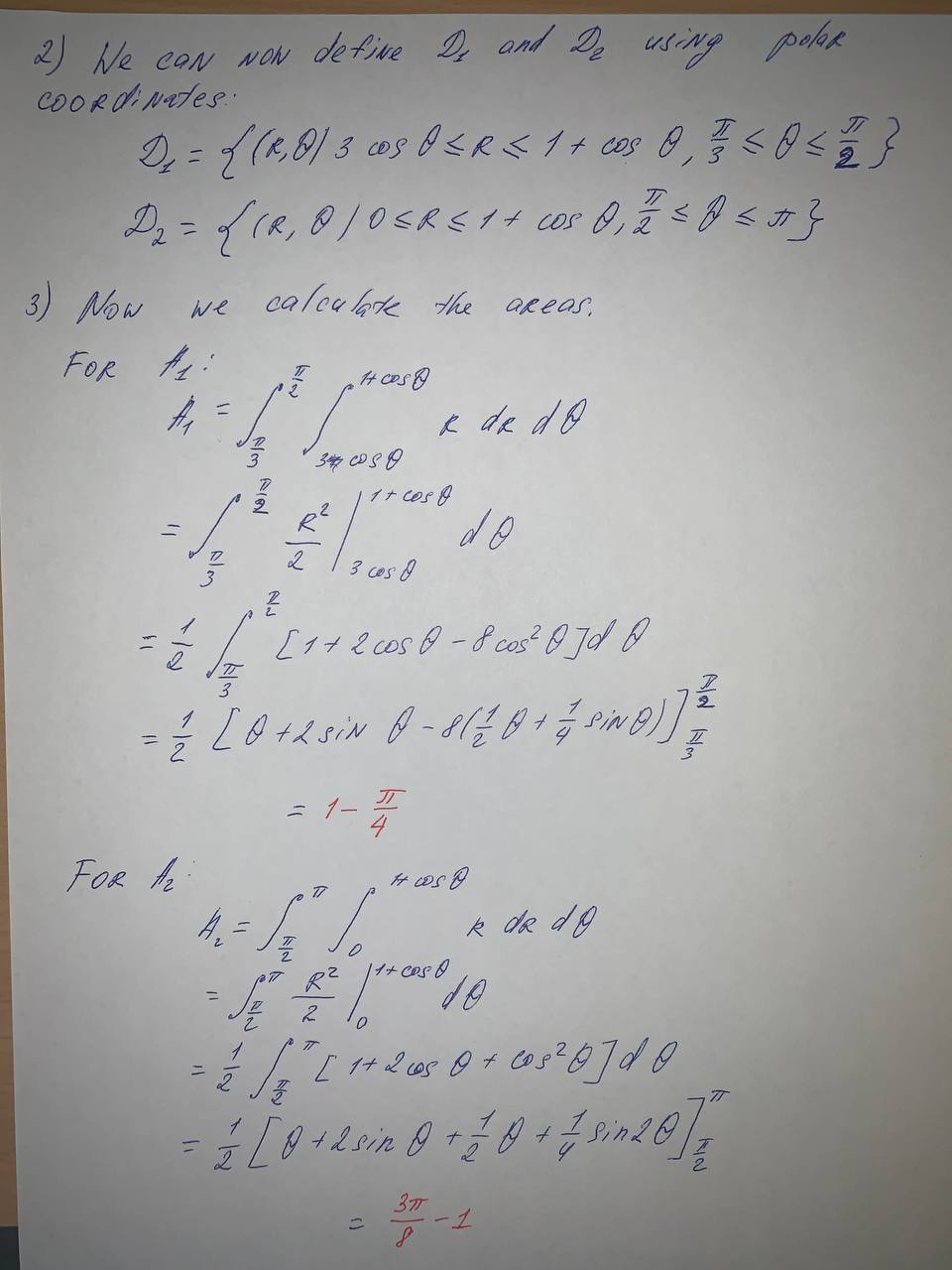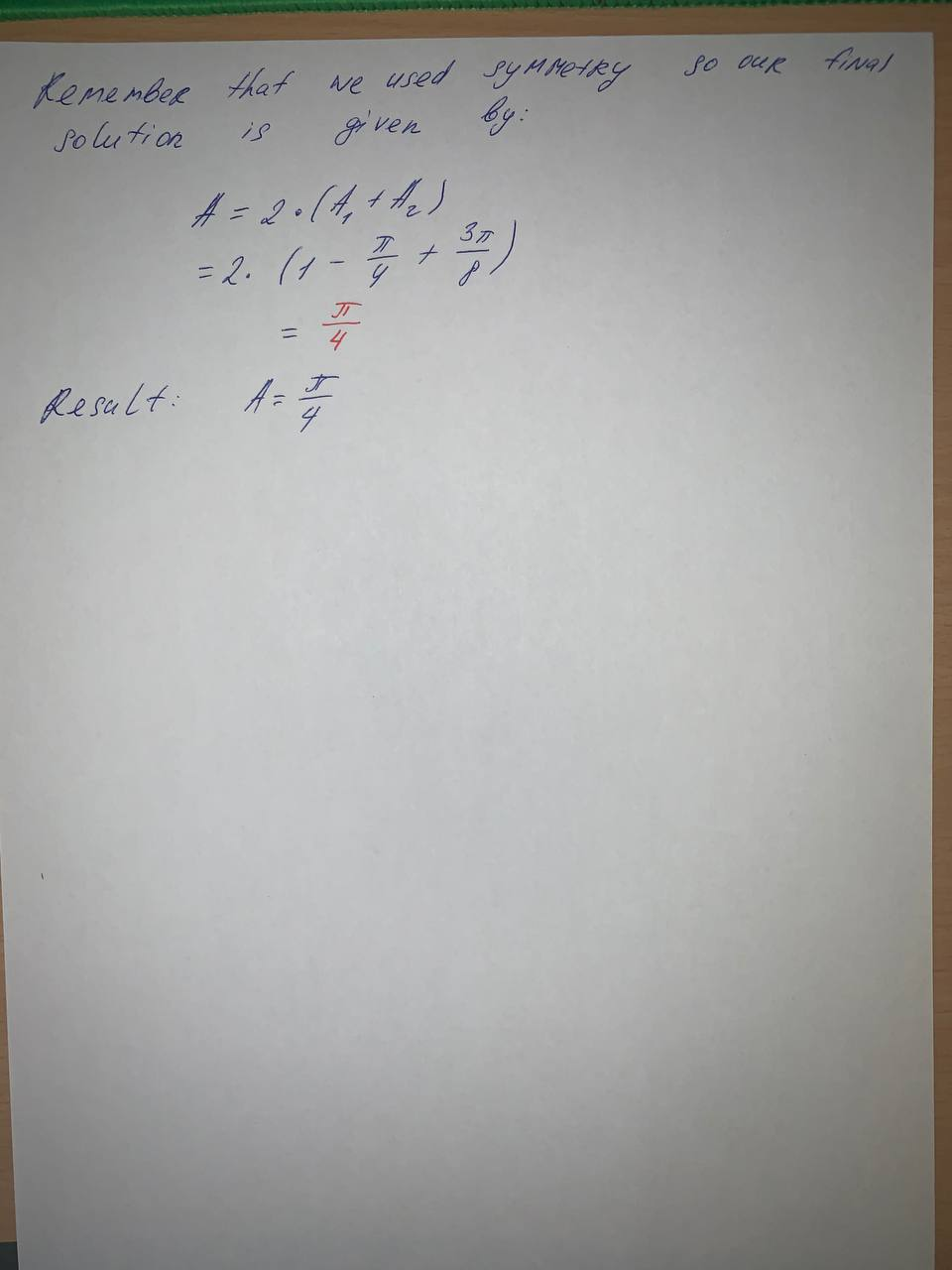Question# Use a double integral to find the area of the region. The region inside the cardioid r=1+\cos \theta and outside the circle r=3 \cos \theta

Integrals
ANSWEREDUse a double integral to find the area of the region. The region inside the cardioid $$r=1+\cos \theta$$ and outside the circle $$r=3 \cos \theta$$2021-06-12...........................